Updating search results...

# 10 Results

View
Selected filters:
• MCCRS.Math.Content.7.NS.A.2d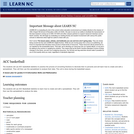Conditional Remix & Share Permitted
CC BY-NC-SA
Rating
0.0 stars

The students will use ACC basketball statistics to practice the process of converting fractions to decimals then to percents and will learn how to create and edit a spreadsheet. They will then use this spreadsheet to analyze their data. This unit is done during the basketball season which takes approximately 15 weeks from the middle of November to the middle of March. Teachers must have Clarisworks to open the sample spreadsheet in the lesson, but may recreate it in another spreadsheet program.

Subject:
Mathematics
Statistics and Probability
Material Type:
Lesson Plan
Provider:
University of North Carolina at Chapel Hill School of Education
Provider Set:
LEARN NC Lesson Plans
Author:
Susan Dougherty
07/14/2000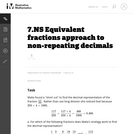Unrestricted Use
CC BY
Rating
0.0 stars

The purpose of the task is to get students to reflect on the definition of decimals as fractions (or sums of fractions), at a time when they are seeing them primarily as an extension of the base-ten number system and may have lost contact with the basic fraction meaning. Students also have their understanding of equivalent fractions and factors reinforced.

Subject:
Mathematics
Material Type:
Activity/Lab
Provider:
Illustrative Mathematics
Provider Set:
Illustrative Mathematics
Author:
Illustrative Mathematics
11/11/2012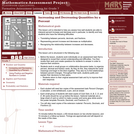Only Sharing Permitted
CC BY-NC-ND
Rating
0.0 stars

This lesson unit is intended to help teachers assess how well students are able to interpret percent increase and decrease, and in particular, to identify and help students who have the following difficulties: translating between percents, decimals, and fractions; representing percent increase and decrease as multiplication; and recognizing the relationship between increases and decreases.

Subject:
Mathematics
Ratios and Proportions
Material Type:
Assessment
Lesson Plan
Provider:
Shell Center for Mathematical Education
Provider Set:
Mathematics Assessment Project (MAP)
04/26/2013Conditional Remix & Share Permitted
CC BY-NC
Rating
0.0 stars

Four full-year digital course, built from the ground up and fully-aligned to the Common Core State Standards, for 7th grade Mathematics. Created using research-based approaches to teaching and learning, the Open Access Common Core Course for Mathematics&nbsp;is designed with student-centered learning in mind, including activities for students to develop valuable 21st century skills and academic mindset.

Subject:
Mathematics
Material Type:
Full Course
Provider:
Pearson
10/06/2016Conditional Remix & Share Permitted
CC BY-NC
Rating
0.0 stars

Working With Rational Numbers

Type of Unit: Concept

Prior Knowledge

Students should be able to:

Compare and order positive and negative numbers and place them on a number line.
Understand the concepts of opposites absolute value.

Lesson Flow

The unit begins with students using a balloon model to informally explore adding and subtracting integers. With the model, adding or removing heat represents adding or subtracting positive integers, and adding or removing weight represents adding or subtracting negative integers.

Students then move from the balloon model to a number line model for adding and subtracting integers, eventually extending the addition and subtraction rules from integers to all rational numbers. Number lines and multiplication patterns are used to find products of rational numbers. The relationship between multiplication and division is used to understand how to divide rational numbers. Properties of addition are briefly reviewed, then used to prove rules for addition, subtraction, multiplication, and division.

This unit includes problems with real-world contexts, formative assessment lessons, and Gallery problems.

Subject:
Algebra
Mathematics
Material Type:
Unit of Study
Provider:
Pearson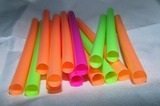Conditional Remix & Share Permitted
CC BY-NC
Rating
0.0 stars

Subject:
Numbers and Operations
Material Type:
Lesson Plan
09/21/2015Conditional Remix & Share Permitted
CC BY-NC
Rating
0.0 stars

Students critique and improve their work on the Self Check. They then extend their knowledge with additional problems.Students solve problems that require them to apply their knowledge of multiplying and dividing positive and negative numbers. Students will then take a quiz.Key ConceptsTo solve the problems in the Self Check, students must apply their knowledge of multiplication and division of positive and negative numbers learned throughout the unit.Goals and Learning ObjectivesUse knowledge of multiplication and division of positive and negative numbers to solve problems.

Subject:
Numbers and Operations
Material Type:
Lesson Plan
09/21/2015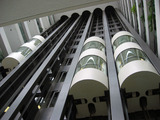Conditional Remix & Share Permitted
CC BY-NC
Rating
0.0 stars

Lesson OverviewStudents learn the definition of rational number, and they write rational numbers as ratios of integers and as repeating or terminating decimals.Key ConceptsStudents have been working with rational numbers throughout this unit, but the term rational number is formally defined in this lesson. A rational number is a number that can be written in the form pq, where p and q are integers. All the integers, fractions, decimals, and percents students have worked with so far in their math classes are rational numbers. Following are some rational numbers written as ratios of integers:36=361−1.2=−12105%=5100 −12=−12Any rational number can also be written as a decimal that terminates or that repeats forever in a regular pattern. For example, 35 = 0.6 and 711 = 0.63636363&hellip; Repeating decimals are often written with a bar over the digits that repeat. For example, 0.63636363&hellip; can be written as 0.63¯.There are numbers that are irrational. These numbers include &pi; and the square root of any whole number that is not a perfect square, such as 2. The decimal form of an irrational number does not terminate, and the digits do not follow a repeating pattern. Students will study irrational numbers in Grade 8.Goals and Learning ObjectivesUnderstand the definition of rational number.Write rational numbers as ratios of integers.Write rational numbers as terminating or repeating decimals.SWD: Students with disabilities may have difficulty working with decimals and fractions, especially moving between the two. If students demonstrate difficulty to the point of frustration, provide direct instruction on the basics for finding equivalent fractions and decimals.ELL: Target and model key language and vocabulary. Specifically, focus on the term rational, as well as terms such as terminate. As you&rsquo;re discussing the key points, write the words on the board or on large sheets of paper and explain/demonstrate what the words mean. Since these are important points that students will be using throughout the module, write them on large poster board so that students can use them as a reference. Have students record new terms, definitions, and examples in their Notebook.&nbsp;

Subject:
Numbers and Operations
Material Type:
Lesson Plan
09/21/2015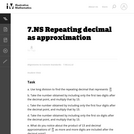Unrestricted Use
CC BY
Rating
0.0 stars

The purpose of the task is to have students reflect on the meaning of repeating decimal representation through approximation. A formal explanation requires the idea of a limit to be made precise, but 7th graders can start to wrestle with the ideas and get a sense of what we mean by an "infinite decimal."

Subject:
Mathematics
Material Type:
Activity/Lab
Provider:
Illustrative Mathematics
Provider Set:
Illustrative Mathematics
Author:
Illustrative Mathematics
01/01/2013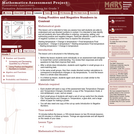Only Sharing Permitted
CC BY-NC-ND
Rating
0.0 stars

This lesson unit is intended to help teachers assess how well students are able to understand and use directed numbers in context. It is intended to help identify and aid students who have difficulties in ordering, comparing, adding, and subtracting positive and negative integers. Particular attention is paid to the use of negative numbers on number lines to explore the structures: starting temperature + change in temperature = final temperature final temperature Đ change in temperature = starting temperature final temperature Đ starting temperature = change in temperature.

Subject:
Mathematics
Numbers and Operations
Material Type:
Assessment
Lesson Plan
Provider:
Shell Center for Mathematical Education
Provider Set:
Mathematics Assessment Project (MAP)